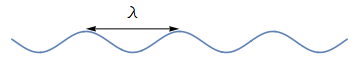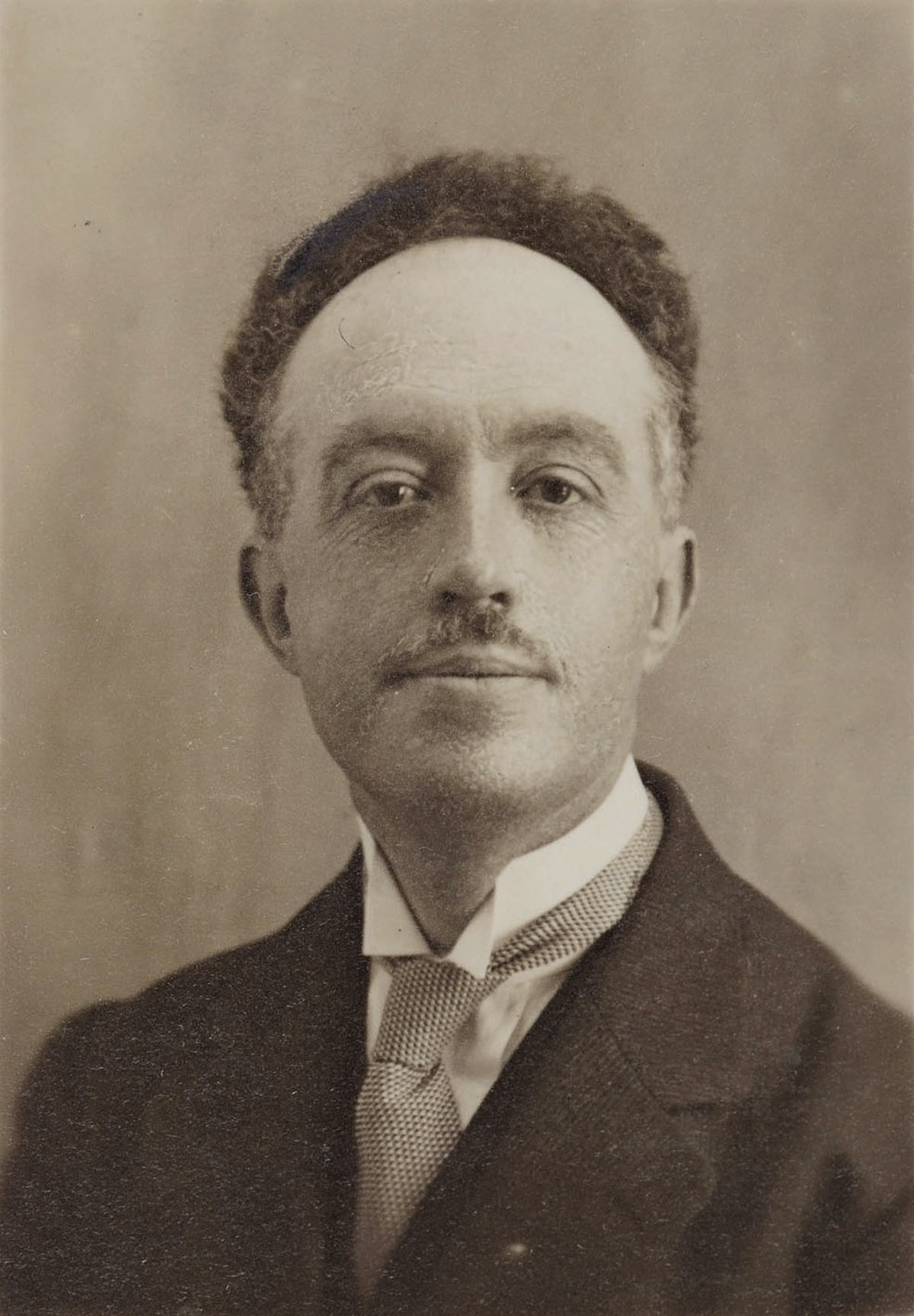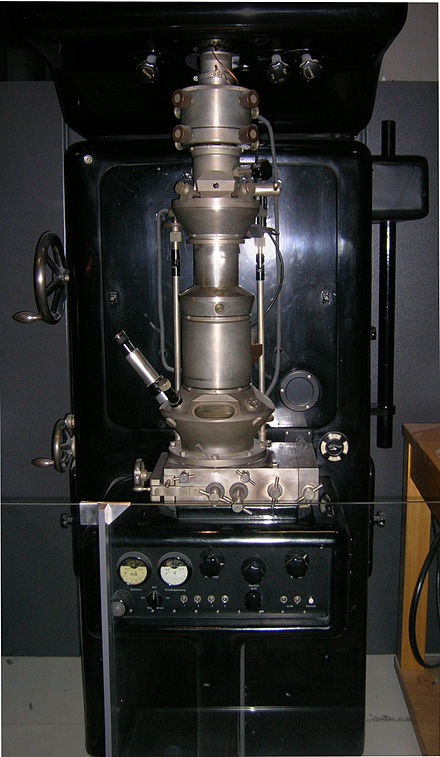## Quantum Mechanics

1.   Everything is a wave
1.2   The wavelength of an electron

### Electromagnetic waves

In any wave, the distance from one crest to the next is called the wavelength. The wavelength is usually denoted by the symbol $$\lambda$$.

First consider electromagnetic waves. Electromagnetic waves can have any wavelength $$\lambda$$. For different ranges of the wavelength we give these waves different names: radio waves, infra red, visible light, etc.

But these names are just for practical convenience; at a fundamental level we just have one kind of electromagnetic wave, with wavelength $$\lambda$$ ranging from zero to infinity.

### The de Broglie relation

Electron waves can also have any wavelength $$\lambda$$. It turns out that this wavelength depends on how much momentum the electron carries.

So we first find the momentum $$p$$ of the electron; this is given by its mass $$m$$ times its velocity $$v$$:

$p~=~ m\, v$

Then the wavelength $$\lambda$$ is given by

$\lambda =\frac{h}{p}$

Here $$h$$ is Planck's constant, with the value in SI units

$\require{color}\colorbox{yellow} {h ~=~ 6.6 \times 10^{-34} \,\, Kg\, m^2/s }$

This formula for $$\lambda$$ is called the de Broglie relation, and $$\lambda$$ is called the de Broglie wavelength of the electron.

From the de Broglie relation we see that slowly moving electrons have a large wavelength, and fast moving electrons have a short wavelength.

### An example

In old days radios contained vacuum tubes that generated and speeded up electrons. A tube operating at 100 Volts would typically speed a electron to a velocity $$v= 10^6\, m/s$$. The mass of the electron is $\require{color}\colorbox{yellow} {m ~=~ 9.1 \times 10^{-31} \,\, Kg }$ From the de Broglie relation we get a wavelength $$\lambda\approx 10^{-10}\, m$$, which is about the size of an atom.

This is why we can use electron microscopes to directly probe the structure of atoms in a crystal. In contrast, visible light has a wavelength which is about 5000 times larger. So with optical microscopes we cannot see the atomic structure of materials.The distance from crest to crest is called the wavelength of the wave.In his1924 Ph.D. thesis, Louis de Broglie postulated that particles like electrons were also waves, and gave a formula for the wavelength.Electron microscope constructed by Ernst Ruska in 1933.